# Optimizing Life Using the XS Performance ProfilerA great way to learn how to use the Moddable SDK's performance profiler effectively to optimize your code is to see how other developers use it to optimize their code. In this article, you'll see how I adapted and optimized JavaScript code written for the web to run well on an ESP32 embedded microcontroller. I used the classic "Game of Life" simulation for this project. Spoiler: the performance improves by nearly 10x.

While every optimization situation is different, there are some common techniques. Here are some of the techniques you'll learn in this article:

• Evaluating the results of a performance profile session to find hotspots
• Streamlining logic
• Validating that code reorganizations don't hurt performance
• Measuring the effectiveness of optimizations
• Using native C strategically to boost performance
• Accelerating bulk operations using JavaScript built-ins

This profiling experiment started with an article I read on Medium: Building Conway’s Game of Life in Javascript [sic]. The cell pattern used in my example is taken from the interesting lexicon at https://playgameoflife.com.

## Version 1

I started by adapting the code of the Medium article to build a Commodetto application.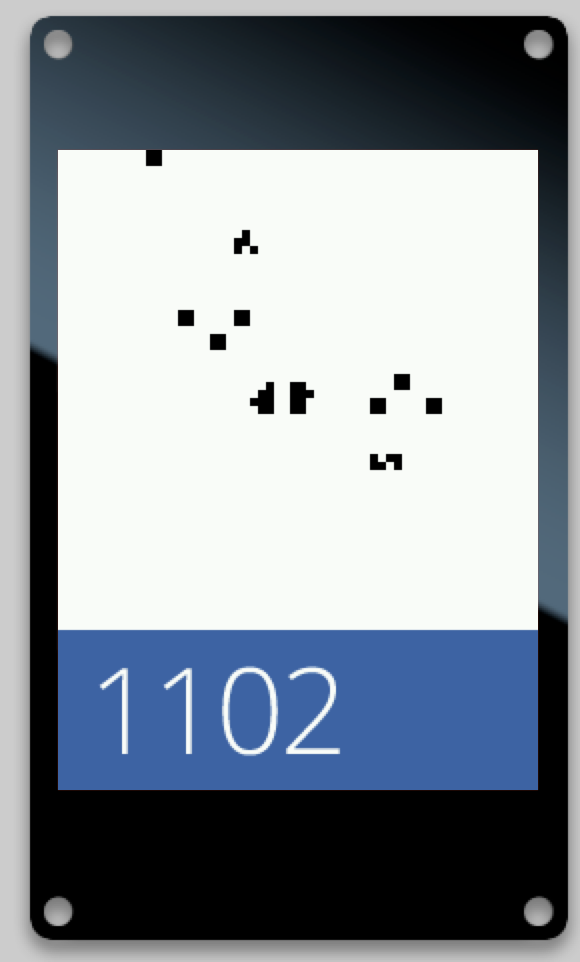The screen displays the cells at the top. The big number at the bottom is the time it took in milliseconds to draw the last frame.

The code of the article uses an array of `Array` objects to store the cells (`1` if alive, `0` if dead). That did not fit in memory on my Moddable Two so I used an array of `Uint8Array` objects instead.

To make the profile easier to read, I changed the arrow functions that draw and compute rows and cells into plain functions.

The core of the algorithm is the `countNeighbors` function which counts the live cells surrounding a cell. That function is called for each cell at every step.

``````function countNeighbors(y, x) {
let count = 0;
for (let i = -1; i < 2; i++) { //This checks the row above and row below
if (x + i >= 0 && x + i < gridSize - 1) { // Check for valid column
if (y > 0 && grid[y - 1][x + i] == 1) {
count++;
}
if (y < gridSize - 1 && grid[y + 1][x + i] == 1) {
count++;
}
}
}
if (x - 1 >= 0 && grid[y][x - 1] == 1) { // Check left cell
count++;
}
if (x + 1 < gridSize - 1 && grid[y][x + 1] == 1) { // Check right cell
count++;
}
return count;
}``````

I got about 790 ms between steps. As expected, the profile showed that the `countNeighbors` function was the most significant hit.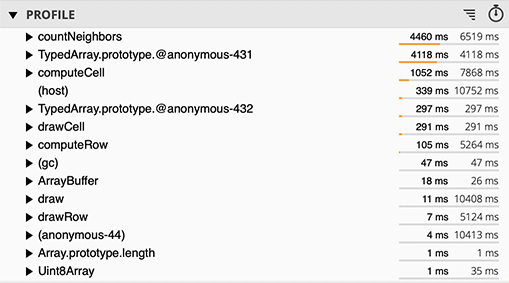`TypedArray.prototype@anonymous-431` and `TypedArray.prototype@anonymous-432` are internal functions used by XS to get and set values in the `Uint8Array` objects.

Full JavaScript code, version 1 ``` import Timer from "timer"; import parseBMP from "commodetto/parseBMP"; import Poco from "commodetto/Poco"; import Resource from "Resource"; const gridSize = 60; let render = new Poco(screen, { displayListLength:13*gridSize*gridSize }); let black = render.makeColor(0, 0, 0); let blue = render.makeColor(49, 101, 173); let white = render.makeColor(255, 255, 255); let grid = new Array(gridSize).fill(); for (let i = 0; i < gridSize; i++) { grid[i] = new Uint8Array(gridSize).fill(0); } const y = 20 const x = 15 grid[y + 0][x + 0] = 1; grid[y + 0][x + 1] = 1; grid[y + 0][x + 7] = 1; grid[y + 0][x + 8] = 1; grid[y + 1][x + 1] = 1; grid[y + 1][x + 1] = 1; grid[y + 1][x + 7] = 1; grid[y + 1][x + 8] = 1; grid[y + 3][x + 4] = 1; grid[y + 3][x + 5] = 1; grid[y + 4][x + 4] = 1; grid[y + 4][x + 5] = 1; grid[y + 9][x + 22] = 1; grid[y + 9][x + 23] = 1; grid[y + 9][x + 25] = 1; grid[y + 9][x + 26] = 1; grid[y + 10][x + 21] = 1; grid[y + 10][x + 27] = 1; grid[y + 11][x + 21] = 1; grid[y + 11][x + 28] = 1; grid[y + 11][x + 31] = 1; grid[y + 11][x + 32] = 1; grid[y + 12][x + 21] = 1; grid[y + 12][x + 22] = 1; grid[y + 12][x + 23] = 1; grid[y + 12][x + 27] = 1; grid[y + 12][x + 31] = 1; grid[y + 12][x + 32] = 1; grid[y + 13][x + 26] = 1; grid[y + 18][x + 24] = 1; grid[y + 18][x + 26] = 1; grid[y + 18][x + 27] = 1; grid[y + 19][x + 24] = 1; grid[y + 19][x + 25] = 1; grid[y + 19][x + 27] = 1; function countNeighbors(y, x) { let count = 0; for (let i = -1; i < 2; i++) { //This checks the row above and row below if (x + i >= 0 && x + i < gridSize - 1) { // Check for valid column if (y > 0 && grid[y - 1][x + i] == 1) { count++; } if (y < gridSize - 1 && grid[y + 1][x + i] == 1) { count++; } } } if (x - 1 >= 0 && grid[y][x - 1] == 1) { // Check left cell count++; } if (x + 1 < gridSize - 1 && grid[y][x + 1] == 1) { // Check right cell count++; } return count; } function draw() { const cellSize = 4; function drawRow(row, y) { function drawCell(alive, x) { if (alive) render.fillRectangle(black, x * cellSize, y * cellSize, cellSize, cellSize); } row.forEach(drawCell); } grid.forEach(drawRow); let newGrid = []; function computeRow(row, y) { let newRow = new Uint8Array(gridSize).fill(0); function computeCell(alive, x) { let count = countNeighbors(y, x); if (alive == 1 && count < 2) { // If alive and < 2 live neighbors alive = 0; } else if (alive == 1 && count > 3) { // If alive and > 3 live neighbors alive = 0; } else if (alive == 0 && count == 3) { // If dead and 3 live neighbors alive = 1; } newRow[x] = alive; }; row.forEach(computeCell); newGrid.push(newRow); } grid.forEach(computeRow); grid = newGrid; } let digits = parseBMP(new Resource("digits-alpha.bmp")); const digitWidth = (digits.width / 10); const digitHeight = digits.height; let when = Date.now(); Timer.repeat(id => { let now = Date.now(); let delta = now - when; when = now; render.begin(); render.fillRectangle(white, 0, 0, 240, 240); draw(); render.fillRectangle(blue, 0, 240, 240, 80); let x = 16; render.drawGray(digits, white, x, 256, Math.floor(delta / 1000) * digitWidth, 0, digitWidth, digitHeight); x += digitWidth; render.drawGray(digits, white, x, 256, Math.floor((delta % 1000) / 100) * digitWidth, 0, digitWidth, digitHeight); x += digitWidth; render.drawGray(digits, white, x, 256, Math.floor((delta % 100) / 10) * digitWidth, 0, digitWidth, digitHeight); x += digitWidth; render.drawGray(digits, white, x, 256, (delta % 10) * digitWidth, 0, digitWidth, digitHeight); render.end(); }, 1); ```

## Version 2

In the original code, the grid is iterated over twice: once to draw and once to compute the next step. I changed that to use the same function to draw and compute rows and cells.

The original also created a new grid for every step. I modified the code to create two grids initially and swap the grids at every step. This saves time allocating the grids and eliminates the need to garbage collect them.

No significant change in performance, but the profile is more readable. That is a sign that the application has been simplified.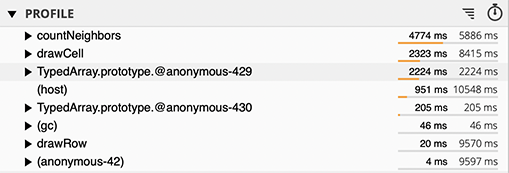Updated JavaScript code, version 2 ``` function countNeighbors(y, x) { let count = 0; for (let i = -1; i < 2; i++) { //This checks the row above and row below if (x + i >= 0 && x + i < gridSize - 1) { // Check for valid column if (y > 0 && grid[y - 1][x + i] == 1) { count++; } if (y < gridSize - 1 && grid[y + 1][x + i] == 1) { count++; } } } if (x - 1 >= 0 && grid[y][x - 1] == 1) { // Check left cell count++; } if (x + 1 < gridSize - 1 && grid[y][x + 1] == 1) { // Check right cell count++; } return count; } function draw() { const cellSize = 4; function drawRow(row, y) { let newRow = newGrid[y]; function drawCell(alive, x) { render.fillRectangle(alive == 1 ? black : white, x * cellSize, y * cellSize, cellSize, cellSize); let count = countNeighbors(y, x); if (alive == 1 && count < 2) { // If alive and < 2 live neighbors alive = 0; } else if (alive == 1 && count > 3) { // If alive and > 3 live neighbors alive = 0; } else if (alive == 0 && count == 3) { // If dead and 3 live neighbors alive = 1; } newRow[x] = alive; } row.forEach(drawCell); } grid.forEach(drawRow); const tmp = grid; grid = newGrid; newGrid = tmp; } ```

## Version 3

The garbage collector (`(gc)` in the profile) was still called because new `Function` objects were created at every step for the sake of arrow function passed to `Array.prototype.forEach` and `TypedArray.prototype.forEach`.

I modified the main loop to use two `for` statements instead of the two `forEach` calls.

I got about 600 ms between steps. `(gc)` no longer appears in the profile. The overhead related to the `drawRow` and `drawCell` functions was gone.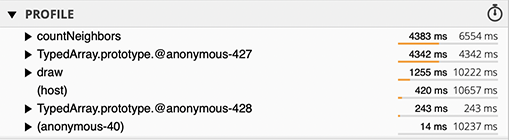Updated JavaScript code, version 3 ``` function countNeighbors(y, x) { let count = 0; for (let i = -1; i < 2; i++) { //This checks the row above and row below if (x + i >= 0 && x + i < gridSize - 1) { // Check for valid column if (y > 0 && grid[y - 1][x + i] == 1) { count++; } if (y < gridSize - 1 && grid[y + 1][x + i] == 1) { count++; } } } if (x - 1 >= 0 && grid[y][x - 1] == 1) { // Check left cell count++; } if (x + 1 < gridSize - 1 && grid[y][x + 1] == 1) { // Check right cell count++; } return count; } function draw() { const cellSize = 4; for (let y = 0; y < gridSize; y++) { const row = grid[y]; const newRow = newGrid[y]; for (let x = 0; x < gridSize; x++) { let alive = row[x]; if (alive) render.fillRectangle(black, x * cellSize, y * cellSize, cellSize, cellSize); let count = countNeighbors(y, x); if (alive == 1 && count < 2) { // If alive and < 2 live neighbors alive = 0; } else if (alive == 1 && count > 3) { // If alive and > 3 live neighbors alive = 0; } else if (alive == 0 && count == 3) { // If dead and 3 live neighbors alive = 1; } newRow[x] = alive; } } const tmp = grid; grid = newGrid; newGrid = tmp; } ```

## Version 4

To go further, I tried to change the representation of the grid. Instead of an array of `Uint8Array` objects I used only one `Uint8Array` object. Cells are accessed by multiplying `y` by the row length and adding `x`.

So the `countNeighbors` function has more operations to do, but the main loop can just increment an offset to access the cell.

As I expected, there were no significant changes, neither in performance nor in the profile. However, the code is now organized in a way that sets the stage for the next optimization. Here profiling is also a way to verify that structural changes to the code do not decrease performance.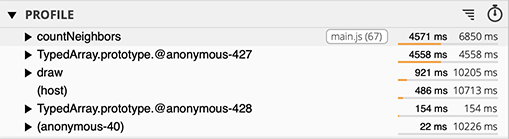Updated JavaScript code, version 4 ``` function countNeighbors(y, x) { let count = 0; for (let i = -1; i < 2; i++) { //This checks the row above and row below if (x + i >= 0 && x + i < gridSize - 1) { // Check for valid column if (y > 0 && grid[((y - 1) * gridSize) + (x + i)] == 1) { count++; } if (y < gridSize - 1 && grid[((y + 1) * gridSize) + (x + i)] == 1) { count++; } } } if (x - 1 >= 0 && grid[((y) * gridSize) + (x - 1)] == 1) { // Check left cell count++; } if (x + 1 < gridSize - 1 && grid[((y) * gridSize) + (x + 1)] == 1) { // Check right cell count++; } return count; } function draw() { const cellSize = 4; let current = 0; for (let y = 0; y < gridSize; y++) { for (let x = 0; x < gridSize; x++) { let alive = grid[current]; if (alive) render.fillRectangle(black, x * cellSize, y * cellSize, cellSize, cellSize); let count = countNeighbors(y, x); if (alive == 1 && count < 2) { // If alive and < 2 live neighbors alive = 0; } else if (alive == 1 && count > 3) { // If alive and > 3 live neighbors alive = 0; } else if (alive == 0 && count == 3) { // If dead and 3 live neighbors alive = 1; } newGrid[current] = alive; current++; } } const tmp = grid; grid = newGrid; newGrid = tmp; } ```

## Version 5

It is time to focus on the `countNeighbors` function, like the profiles indicated. The new representation of the grid allowed me to streamline the code by caching offsets and unrolling loops.

``````function countNeighbors(y, x) {
const offset = (y * gridSize) + x;
const limit = gridSize - 1;
let count = 0;
if (y > 0) {
const tmp = offset - gridSize;
if (x > 0) {
if (grid[tmp - 1])
count++;
}
if (grid[tmp])
count++;
if (x < limit) {
if (grid[tmp + 1])
count++;
}
}
if (x > 0) {
if (grid[offset - 1])
count++;
}
if (x < limit) {
if (grid[offset + 1])
count++;
}
if (y < limit) {
const tmp = offset + gridSize;
if (x > 0) {
if (grid[tmp - 1])
count++;
}
if (grid[tmp])
count++;
if (x < limit) {
if (grid[tmp + 1])
count++;
}
}
return count;
}``````

I got about 440 ms between steps, which is about twice as fast as the initial version, already a significant improvement.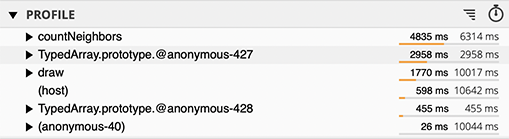Updated JavaScript code, version 5 ``` function countNeighbors(y, x) { const offset = (y * gridSize) + x; const limit = gridSize - 1; let count = 0; if (y > 0) { const tmp = offset - gridSize; if (x > 0) { if (grid[tmp - 1]) count++; } if (grid[tmp]) count++; if (x < limit) { if (grid[tmp + 1]) count++; } } if (x > 0) { if (grid[offset - 1]) count++; } if (x < limit) { if (grid[offset + 1]) count++; } if (y < limit) { const tmp = offset + gridSize; if (x > 0) { if (grid[tmp - 1]) count++; } if (grid[tmp]) count++; if (x < limit) { if (grid[tmp + 1]) count++; } } return count; } function draw() { const cellSize = 4; let current = 0; for (let y = 0; y < gridSize; y++) { for (let x = 0; x < gridSize; x++) { let alive = grid[current]; if (alive) render.fillRectangle(black, x * cellSize, y * cellSize, cellSize, cellSize); let count = countNeighbors(y, x); if (alive == 1 && count < 2) { // If alive and < 2 live neighbors alive = 0; } else if (alive == 1 && count > 3) { // If alive and > 3 live neighbors alive = 0; } else if (alive == 0 && count == 3) { // If dead and 3 live neighbors alive = 1; } newGrid[current] = alive; current++; } } const tmp = grid; grid = newGrid; newGrid = tmp; } ```

## Version 6

Now that the `countNeighbors` function was simplified, it is easy enough to implement in C.

``function countNeighbors(gridBuffer, gridSize, y, x) @ "xs_countNeighbors";``

I got about 140 ms between steps! And the `countNeighbors` function disappeared from the profile.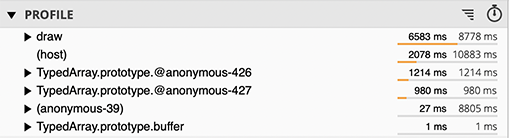Updated JavaScript code, version 6 ``` function countNeighbors(gridBuffer, gridSize, y, x) @ "xs_countNeighbors"; function draw() { const cellSize = 4; let current = 0; for (let y = 0; y < gridSize; y++) { for (let x = 0; x < gridSize; x++) { let alive = grid[current]; if (alive) render.fillRectangle(black, x * cellSize, y * cellSize, cellSize, cellSize); let count = countNeighbors(y, x); if (alive == 1 && count < 2) { // If alive and < 2 live neighbors alive = 0; } else if (alive == 1 && count > 3) { // If alive and > 3 live neighbors alive = 0; } else if (alive == 0 && count == 3) { // If dead and 3 live neighbors alive = 1; } newGrid[current] = alive; current++; } } const tmp = grid; grid = newGrid; newGrid = tmp; } ```
C code ``` #include "xsmc.h" void xs_countNeighbors(xsMachine *the) { uint8_t* gridBuffer = xsmcToArrayBuffer(xsArg(0)); xsIntegerValue gridSize = xsmcToInteger(xsArg(1)); xsIntegerValue y = xsmcToInteger(xsArg(2)); xsIntegerValue x = xsmcToInteger(xsArg(3)); xsIntegerValue offset = (y * gridSize) + x; xsIntegerValue limit = gridSize - 1; xsIntegerValue count = 0; if (y > 0) { if (x > 0) { if (gridBuffer[offset - gridSize - 1]) count++; } if (gridBuffer[offset - gridSize]) count++; if (x < limit) { if (gridBuffer[offset - gridSize + 1]) count++; } } if (x > 0) { if (gridBuffer[offset - 1]) count++; } if (x < limit) { if (gridBuffer[offset + 1]) count++; } if (y < limit) { if (x > 0) { if (gridBuffer[offset + gridSize - 1]) count++; } if (gridBuffer[offset + gridSize]) count++; if (x < limit) { if (gridBuffer[offset + gridSize + 1]) count++; } } xsmcSetInteger(xsResult, count); } ```

The option to implement functions in native C is a feature of embedded programming in JavaScript using XS, an option that isn't available to JavaScript developers on the web. C should be used sparingly, where it can be most effective. The performance profiler in XS gives you insight into which functions would benefit the most from being implemented in C. Moving a function from JavaScript to C doesn't change any of the code that calls it. As you can see, calling the native C version is exactly the same as calling the original JavaScript version.

## Version 7

With `countNeighbors` under control, the `draw` function now uses the most time. Revisiting its implementation, there are two opportunities for optimization:

• Some conditions are checked more than once. By rearranging the logic, the number of conditionals for each cell is reduced.
• On each step, most grid cells stay the same. Instead of copying the previous value of each grid cell individually, it is more efficient to copy them as a bulk operation. In this case, `Uint8Array`'s `set` can be used to copy the previous state of all grid cells, and then the script only needs to set the cells that change their state.

With these two changes, I got about 90 ms between steps. That's almost 10 times faster than the original. Notice that `anonymous-427`, the `Uint8Array` setter, now uses much less time because it is called infrequently.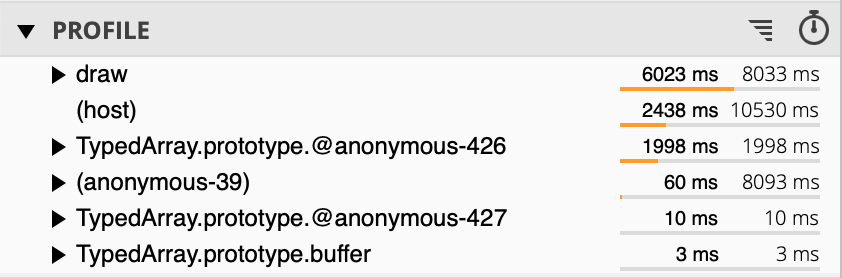Updated JavaScript code, version 7 ``` function countNeighbors(gridBuffer, gridSize, y, x) @ "xs_countNeighbors"; function draw() { const gridBuffer = grid.buffer; const cellSize = 4; let current = 0; newGrid.set(grid); for (let y = 0; y < gridSize; y++) { for (let x = 0; x < gridSize; x++) { let count = countNeighbors(gridBuffer, gridSize, y, x); if (grid[current]) { render.fillRectangle(black, x * cellSize, y * cellSize, cellSize, cellSize); if (count < 2) // If alive and < 2 live neighbors newGrid[current] = 0; else if (count > 3) // If alive and > 3 live neighbors newGrid[current] = 0; } else if (count == 3) // If dead and 3 live neighbors newGrid[current] = 1; current++; } } const tmp = grid; grid = newGrid; newGrid = tmp; } ```

## Conclusion

Performance is often a critical aspect of a product. If a product performs poorly, it may not be viable in the market. By making good use of the performance profiler in XS, huge gains are possible. You can see that in this video that shows the before and after versions of the Game of Life app optimized in this article. The feeling of the two simulations is completely different, simply because one outperforms the other by an order of magnitude.

This article took you step-by-step through how I used the XS performance profiler to optimize the Game of Life simulation. At the start, it may not have seemed possible to improve performance much. By taking an incremental approach, new opportunities for performance improvement appeared. While every performance challenge is unique, the techniques used in this article can help you overcome the performance challenges in your life.